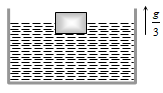#12 | Solved Problems: Set 1
(Physics) > Mechanical Properties of Fluids
Related Practice Questions :

Two bodies are in equilibrium when suspended in water from the arms of a balance. The mass of one body is 36 g and its density is 9 g / cm3. If the mass of the other is 48 g, its density in g / cm3 is
(a)$\frac{4}{3}$                                         (b) $\frac{3}{2}$
(c) 3                                           (d) 5

Complete Question Bank + Test Series
Complete Question Bank

Difficulty Level:

An ice berg of density 900 Kg/${\mathrm{m}}^{3}$ is floating in water of density 1000 Kg/${\mathrm{m}}^{3}$. The percentage of volume of ice-cube outside the water is
(a) 20%                                       (b) 35%
(c) 10%                                       (d) 25%

Complete Question Bank + Test Series
Complete Question Bank

Difficulty Level:

A log of wood of mass 120 Kg floats in water. The weight that can be put on the raft to make it just sink, should be (density of wood = 600 Kg/${\mathrm{m}}^{3}$
(a) 80 Kg                                                 (b) 50 Kg
(c) 60 Kg                                                  (d) 30 Kg

Complete Question Bank + Test Series
Complete Question Bank

Difficulty Level:

A metallic block of density 5 gm ${\mathrm{cm}}^{-3}$ and having dimensions 5 cm × 5 cm × 5 cm is weighed in water. Its apparent weight will be
(a) 5 × 5 × 5 × 5 gf                            (b) 4 × 4 × 4 × 4 gf
(c) 5 × 4 × 4 × 4 gf                            (d) 4 × 5 × 5 × 5 gf

Complete Question Bank + Test Series
Complete Question Bank

Difficulty Level:

A cubical block is floating in a liquid with half of its volume immersed in the liquid. When the whole system accelerates upwards with acceleration of g/3, the fraction of volume immersed in the liquid will be(a) $\frac{1}{2}$                                  (b) $\frac{3}{8}$

(c) $\frac{2}{3}$                                  (d) $\frac{3}{4}$